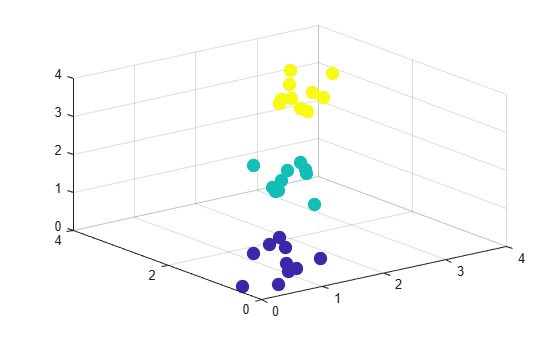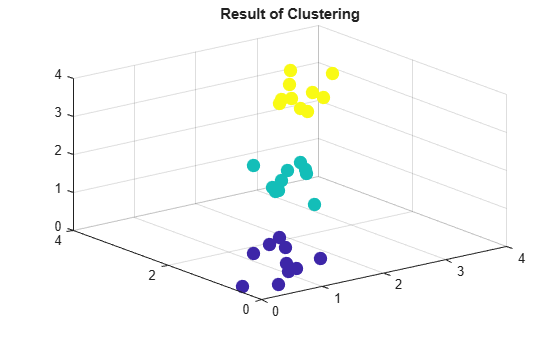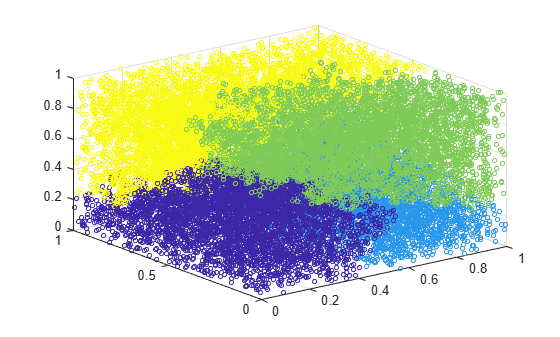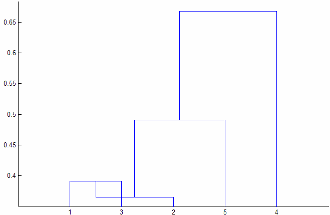# clusterdata

Construct agglomerative clusters from data

## Syntax

``T = clusterdata(X,cutoff)``
``T = clusterdata(X,Name,Value)``

## Description

example

````T = clusterdata(X,cutoff)` returns cluster indices for each observation (row) of an input data matrix `X`, given a threshold `cutoff` for cutting an agglomerative hierarchical tree that the `linkage` function generates from `X`.`clusterdata` supports agglomerative clustering and incorporates the `pdist`, `linkage`, and `cluster` functions, which you can use separately for more detailed analysis. See Algorithm Description for more details.```

example

````T = clusterdata(X,Name,Value)` specifies clustering options using one or more name-value pair arguments. You must specify either `Cutoff` or `MaxClust`. For example, specify `'MaxClust',5` to find a maximum of five clusters.```

## Examples

collapse all

Find and visualize a maximum of three clusters in a randomly generated data set using two different approaches:

1. Specify a value for the `cutoff` input argument.

2. Specify a value for the `'MaxClust'` name-value pair argument.

Create a sample data set consisting of randomly generated data from three standard uniform distributions.

```rng('default'); % For reproducibility X = [gallery('uniformdata',[10 3],12); ... gallery('uniformdata',[10 3],13)+1.2; ... gallery('uniformdata',[10 3],14)+2.5]; y = [ones(10,1);2*(ones(10,1));3*(ones(10,1))]; % Actual classes```

Create a scatter plot of the data.

```scatter3(X(:,1),X(:,2),X(:,3),100,y,'filled') title('Randomly Generated Data in Three Clusters');```Find a maximum of three clusters in the data by specifying the value 3 for the `cutoff` input argument.

`T1 = clusterdata(X,3);`

Because the value of `cutoff` is greater than 2, `clusterdata` interprets `cutoff` as the maximum number of clusters.

Plot the data with the resulting cluster assignments.

```scatter3(X(:,1),X(:,2),X(:,3),100,T1,'filled') title('Result of Clustering');```Find a maximum of three clusters by specifying the value 3 for the `'MaxClust'` name-value pair argument.

`T2 = clusterdata(X,'Maxclust',3); `

Plot the data with the resulting cluster assignments.

```scatter3(X(:,1),X(:,2),X(:,3),100,T2,'filled') title('Result of Clustering');```Using both approaches, `clusterdata` identifies the three distinct clusters in the data.

Create a hierarchical cluster tree and find clusters in one step. Visualize the clusters using a 3-D scatter plot.

Create a 20,000-by-3 matrix of sample data generated from the standard uniform distribution.

```rng('default'); % For reproducibility X = rand(20000,3);```

Find a maximum of four clusters in a hierarchical cluster tree created using the `ward` linkage method. Specify `'SaveMemory'` as `'on'` to construct clusters without computing the distance matrix. Otherwise, you can receive an out-of-memory error if your machine does not have enough memory to hold the distance matrix.

`T = clusterdata(X,'Linkage','ward','SaveMemory','on','Maxclust',4);`

Plot the data with each cluster shown in a different color.

`scatter3(X(:,1),X(:,2),X(:,3),10,T)``clusterdata` identifies four clusters in the data.

## Input Arguments

collapse all

Input data, specified as a numeric matrix with two or more rows. The rows represent observations, and the columns represent categories or dimensions.

Data Types: `single` | `double`

Threshold for cutting the hierarchical tree defined by `linkage`, specified as a positive scalar between `0` and `2` or a positive integer `≥ 2`. `clusterdata` behaves differently depending on the value specified for `cutoff`.

• If `0 < cutoff < 2`, then `clusterdata` forms clusters when `inconsistent` values are greater than `cutoff`.

• If `cutoff` is an integer `≥ 2`, then `clusterdata` forms a maximum of `cutoff` clusters.

When you specify `cutoff`, you cannot specify any name-value pair arguments.

Example: `clusterdata(X,3)`

Data Types: `single` | `double`

### Name-Value Pair Arguments

Specify optional comma-separated pairs of `Name,Value` arguments. `Name` is the argument name and `Value` is the corresponding value. `Name` must appear inside quotes. You can specify several name and value pair arguments in any order as `Name1,Value1,...,NameN,ValueN`.

Example: `clusterdata(X,'Linkage','ward','MaxClust',3)` specifies creating a maximum of three clusters of `X` using Ward linkage.

Criterion for defining clusters in a hierarchical cluster tree, specified as the comma-separated pair consisting of `'Criterion'` and either `'inconsistent'` or `'distance'`. When you specify `'Criterion'`, you must also specify a value for `MaxClust` or `Cutoff`.

Example: `clusterdata(X,'Criterion','distance','Cutoff',.5)`

Data Types: `char` | `string`

Cutoff for inconsistent or distance criterion, specified as the comma-separated pair consisting of `'Cutoff'` and a positive scalar. `clusterdata` uses `Cutoff` as a threshold for either the heights or the inconsistency coefficients of nodes, depending on the value of `Criterion`. If you specify a value for `'Cutoff'` without specifying the criterion for defining clusters, then `clusterdata` uses the `'inconsistent'` criterion by default.

• If `'Criterion'` is `'distance'`, then `clusterdata` groups all leaves at or below a node into a cluster, provided that the height of the node is less than `Cutoff`.

• If `'Criterion'` is `'inconsistent'`, then the `inconsistent` values of a node and all its subnodes must be less than `Cutoff` for `clusterdata` to group them into a cluster.

You must specify either `Cutoff` or `MaxClust`.

Example: `clusterdata(X,'Cutoff',0.2)`

Data Types: `single` | `double`

Depth for computing inconsistent values, specified as the comma-separated pair consisting of `'Depth'` and a numeric scalar. `clusterdata` evaluates inconsistent values by looking to the specified depth below each node in the hierarchical cluster tree. When you specify `'Depth'`, you must also specify a value for `MaxClust` or `Cutoff`.

Example: `clusterdata(X,'Depth',3,'Cutoff',0.5)`

Data Types: `single` | `double`

Distance metric, specified as the comma-separated pair consisting of `'Distance'` and any distance metric accepted by the `pdist` function, as descried in the following table. When you specify `'Distance'`, you must also specify a value for `MaxClust` or `Cutoff`.

MetricDescription
`'euclidean'`

Euclidean distance (default)

`'squaredeuclidean'`

Squared Euclidean distance. (This option is provided for efficiency only. It does not satisfy the triangle inequality.)

`'seuclidean'`

Standardized Euclidean distance. Each coordinate difference between observations is scaled by dividing by the corresponding element of the standard deviation, `S = std(X,'omitnan')`.

`'mahalanobis'`

Mahalanobis distance using the sample covariance of `X`, ```C = cov(X,'omitrows')```

`'cityblock'`

City block distance

`'minkowski'`

Minkowski distance. The default exponent is 2. To use a different exponent `P`, specify `P` after `'minkowski'`, where `P` is a positive scalar value: `'minkowski',P`.

`'chebychev'`

Chebychev distance (maximum coordinate difference)

`'cosine'`

One minus the cosine of the included angle between points (treated as vectors)

`'correlation'`

One minus the sample correlation between points (treated as sequences of values)

`'hamming'`

Hamming distance, which is the percentage of coordinates that differ

`'jaccard'`

One minus the Jaccard coefficient, which is the percentage of nonzero coordinates that differ

`'spearman'`

One minus the sample Spearman's rank correlation between observations (treated as sequences of values)

`@distfun`

Custom distance function handle. A distance function has the form

```function D2 = distfun(ZI,ZJ) % calculation of distance ...```
where

• `ZI` is a `1`-by-`n` vector containing a single observation.

• `ZJ` is an `m2`-by-`n` matrix containing multiple observations. `distfun` must accept a matrix `ZJ` with an arbitrary number of observations.

• `D2` is an `m2`-by-`1` vector of distances, and `D2(k)` is the distance between observations `ZI` and `ZJ(k,:)`.

If your data is not sparse, using a built-in `distance` is generally faster than using a function handle.

Example: `clusterdata(X,'Distance','minkowski','MaxClust',4)`

Data Types: `char` | `string` | `function_handle`

Algorithm for computing distance between clusters, specified as the comma-separated pair consisting of `'Linkage'` and any algorithm accepted by the `linkage` function, as described in the following table. When you specify `'Linkage'`, you must also specify a value for `MaxClust` or `Cutoff`.

AlgorithmDescription
`'average'`

Unweighted average distance (UPGMA)

`'centroid'`

Centroid distance (UPGMC), appropriate for Euclidean distances only

`'complete'`

Farthest distance

`'median'`

Weighted center of mass distance (WPGMC), appropriate for Euclidean distances only

`'single'`

Shortest distance

`'ward'`

Inner squared distance (minimum variance algorithm), appropriate for Euclidean distances only

`'weighted'`

Weighted average distance (WPGMA)

Example: `clusterdata(X,'Linkage','median','MaxClust',4)`

Data Types: `char` | `string`

Maximum number of clusters to form, specified as the comma-separated pair consisting of `'MaxClust'` and a positive integer.

You must specify either `Cutoff` or `MaxClust`.

Example: `clusterdata(X,'MaxClust',4)`

Data Types: `single` | `double`

Option for saving memory, specified as the comma-separated pair consisting of `'SaveMemory'` and either `'on'` or `'off'`. When you specify `'SaveMemory'`, you must also specify a value for `MaxClust` or `Cutoff`.

The `'on'` setting causes `clusterdata` to construct clusters without computing the distance matrix. The `'on'` setting applies when both of these conditions are satisfied:

When these two conditions apply, the default value for `'SaveMemory'` is `'on'` if `X` has 20 columns or fewer, or if the computer does not have enough memory to store the distance matrix. Otherwise, the default value for `'SaveMemory'` is `'off'`.

When `'SaveMemory'` is `'on'`, the `linkage` run time is proportional to the number of dimensions (number of columns of `X`). When `'SaveMemory'` is `'off'`, the `linkage` memory requirement is proportional to `N2`, where `N` is the number of observations. Choosing the best (least-time) setting for `'SaveMemory'` depends on the problem dimensions, number of observations, and available memory. The default `'SaveMemory'` setting is a rough approximation of an optimal setting.

Example: `'SaveMemory','on'`

Data Types: `char` | `string`

## Output Arguments

collapse all

Cluster indices, returned as a numeric column vector. `T` has as many rows as `X`, and each row of `T` indicates the cluster assignment of the corresponding observation in `X`.

## Tips

• If `'Linkage'` is `'centroid'` or `'median'`, then `linkage` can produce a cluster tree that is not monotonic. This result occurs when the distance from the union of two clusters, r and s, to a third cluster is less than the distance between r and s. In this case, in a dendrogram drawn with the default orientation, the path from a leaf to the root node takes some downward steps. To avoid this result, specify another value for `'Linkage'`. The following image shows a nonmonotonic cluster tree.In this case, cluster 1 and cluster 3 are joined into a new cluster, while the distance between this new cluster and cluster 2 is less than the distance between cluster 1 and cluster 3.

## Algorithms

If you specify a value `c` for the `cutoff` input argument, then ```T = clusterdata(X,c)``` performs the following steps:

1. Create a vector of the Euclidean distance between pairs of observations in `X` by using `pdist`.

```Y = pdist(X,'euclidean')```

2. Create an agglomerative hierarchical cluster tree from `Y` by using `linkage` with the `'single'` method for computing the shortest distance between clusters.

`Z = linkage(Y,'single')`

3. If `0 < ``c`` < 2`, use `cluster` to define clusters from `Z` when inconsistent values are less than `c`.

```T = cluster(Z,'Cutoff',c)```

4. If `c` is an integer value `≥ 2`, use `cluster` to find a maximum of `c` clusters from `Z`.

`T = cluster(Z,'MaxClust',c)`

## Alternative Functionality

If you have a hierarchical cluster tree `Z` (the output of the `linkage` function for the input data matrix `X`), you can use `cluster` to perform agglomerative clustering on `Z` and return the cluster assignment for each observation (row) in `X`.